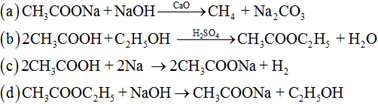# NCERT Exemplar Problems and their Solutions

Reviewed By:
Krishna Kant Majee
M.Sc., B.Ed.

### Chapter 4-Carbon and Its Compounds-MCQs

Multiple Choice Questions (MCQs)
Q.1. Carbon exists in the atmosphere in the form of
(a) Only carbon monoxide
(b) Carbon monoxide in traces and carbon dioxide
(c) Only carbon dioxide
(d) Coal

Explanation: Carbon exists only in the form of carbon dioxide gas $(CO_{2})$ in air.
Q.2. Which of the following statements are usually correct for carbon compounds? These
(i) Are good conductors of electricity.
(ii) Are poor conductors of electricity.
(iii) Have strong forces of attraction between their molecules.
(iv) Do not have strong forces of attraction between their molecules.
(a) (i) and (iii)
(b) (ii) and (iii)
(c) (i) and (iv)
(d) (ii) and (iv)
Explanation: Carbon having four valence electrons forms only covalent compounds which exhibit less intermolecular attractions and do not have any free electrons to carry electric current. Therefore, these are poor conductors of electricity.
Q.3. A molecule of ammonia $(NH_{3})$ has
(a) Only single bonds
(b) Only double bonds
(c) Only triple bonds
(d) Two double bonds and one single bond
Explanation: Ammonia is a covalent molecule in which center nitrogen atom is bonded with three hydrogen atoms through single covalent bond.
Q.4. Buckminsterfullerene is an allotropic form of
(a) Phosphorus
(b) Sulphur
(c) Carbon
(d) Tin
Explanation: Buckminsterfullerene is an allotrope of carbon with 60 carbon atoms which are joined together in a spherical shape.
Q.5. Which of the following are correct structural isomers of $C_{4}H_{10}?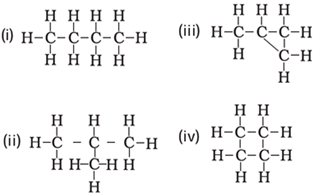(a) (i) and (iii) (b) (ii) and (iv) (c) (i) and (ii) (d) (iii) and (iv) Answer. (a) Explanation: Structural isomers have same molecular formula but different parent chain of carbon atoms in the molecule. Q.6. In the following reaction, alkaline$KMnO_{4}$acts as: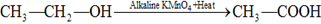(a) Reducing agent (b) Oxidising agent (c) Catalyst (d) Dehydrating agent Answer. (b) Explanation:$KMnO_{4}$acts as oxidising agent as it oxidized$CH_{3}CH_{2}OH$to$CH_{3}COOH$by addition of oxygen atom. Q.7. Oils on treating with hydrogen in the presence of palladium or nickel catalyst form fats. This is an example of (a) Addition reaction (b) Substitution reaction (c) Displacement reaction (d) Oxidation reaction Answer. (a) Explanation: It is hydrogenation reaction means addition of hydrogen to double bonds of unsaturated compounds found in oil.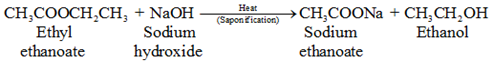Q.8. In which of the following compounds -OH is the functional group? (a) Butanone (b) Butanol (c) Butanoic (d) Butanal Answer. (b) Explanation: Compounds with –OH functional group are ended with suffix –ol.$C_{4}H_{9} ─ OH or CH_{3} ─ CH_{2} ─ CH_{2} ─ CH_{2} ─ OH$Q.9. The soap molecule has a (a) Hydrophilic head and a hydrophobic tail (b) Hydrophobic head and a hydrophilic tail (c) Hydrophobic head and a hydrophobic tail (d) Hydrophilic head and a hydrophilic tail Answer. (a) Explanation: A soap molecule contains a long hydrocarbon part and a small ionic part of -COONa group. Hydrocarbon chain is hydrophobic or water repelling whereas ionic head is hydrophilic or water attracting group.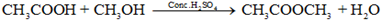Q.10. Which of the following is the correct representation of electron dot structure of nitrogen?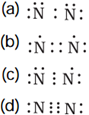Answer. (d) Explanation: Nitrogen molecule is a covalent molecule in which two nitrogen atoms are bonded through triple covalent bond with one lone pair of electrons over each nitrogen atom. Q.11. Structural formula of ethyne is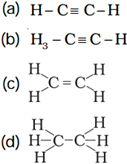Answer. (a) Explanation: General formula for alkyne is C_{n}H_{2n-2}$. There must be at least one triple bond between carbon atoms. With two carbon atom, the possible structure would be H ─ C ≡ C ─ H.
Q.12. Identify the unsaturated compounds from the following.
(i) Propane
(ii) Propene
(iii) Propyne
(iv) Chloropropane
(a) (i) and (ii)
(b) (ii) and (iv)
(c) (iii) and (iv)
(d) (ii) and (iii)
Explanation: Alkene and alkyne are unsaturated hydrocarbon as they have double and triple covalent bonds between carbon atoms.
Q.13. Chlorine reacts with saturated hydrocarbons at room temperature in the
(a) absence of sunlight
(b) presence of sunlight
(c) presence of water
(d) presence of hydrochloric acid
Explanation: Chlorine shows photochemical substitution reactions with saturated hydrocarbons that occurs in the presence of light.

Q.14. In the soap micelles
(a) The ionic end of soap is on the surface of the cluster while the carbon chain is in the interior of the cluster
(b) Ionic end of soap is in the interior of the cluster and the carbon chain is out of the cluster
(c) Both ionic end and carbon chain are in the interior of the cluster
(d) Both ionic end and carbon chain are on the exterior of the cluster
Explanation: A micelle is a spherical aggregation of soap molecules in water in which hydrocarbon ends are directed towards the centre and ionic ends are directed outwards.
Q.15. Pentane has the molecular formula C5H12. It has
(a) 5 covalent bonds
(b) 12 covalent bonds
(c) 16 covalent bonds
(d) 17 covalent bonds
Explanation: Pentane contains four C-C bonds and twelve C-H covalent bonds.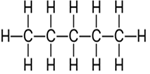Q.16. Structural formula of benzene is: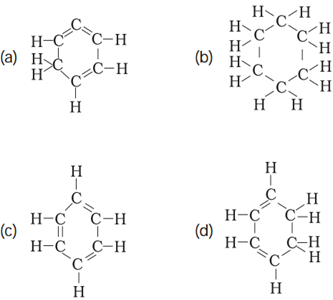Explanation: Benzene is simplest aromatic compound with six carbon atoms and six H atoms. There are three alternate pi bonds in ring of carbon atoms.
Q.17. Ethanol reacts with sodium and forms two products. These are
(a) Sodium ethanoate and hydrogen
(b) Sodium ethanoate and oxygen
(c) Sodium ethoxide and hydrogen
(d) Sodium ethoxide and oxygen
Explanation: Ethanol $(C_{2}H_{5}OH)$ reacts with sodium to form sodium ethoxide (C2H5ONa) along with liberation of hydrogen gas.
$2C_{2}H_{5}OH + 2Na\rightarrow 2C_{2}H_{5}ONa + H_{2}\uparrow$

Q.18. The correct structural formula of butanoic acid is: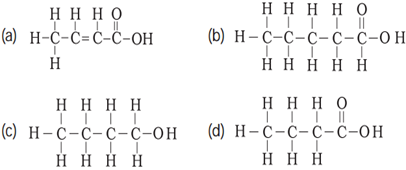Explanation: Butanoic acid is a carboxylic acid with four carbon atom and one –COOH group at terminal.
Q.19. Vinegar is a solution of:
(a) 50% – 60% acetic acid in alcohol
(b) 5% – 8% acetic acid in alcohol
(c) 5% – 8% acetic acid in water
(d) 50%- 60% acetic acid in water
Explanation: Vinegar is a 5%-8% aqueous solution of acetic acid.
Question. 20 Mineral acids are stronger acids than carboxylic acids because
(i) Mineral acids are completely ionised.
(ii) Carboxylic acids are completely ionised.
(iii) Mineral acids are partially ionised.
(iv) Carboxylic acids are partially ionised.
(a) (i) and (iv)
(b) (ii) and (iii)
(c) (i) and (ii)
(d) (iii) and (iv)
Ans. (a)
Explanation: Mineral acids like nitric acid, sulphuric acid are stronger than carboxylic acid as they can ionize 100% in their solution.
Question. 21 Carbon forms four covalent bonds by sharing its four valence electrons with four univalent atoms, e.g. hydrogen. After the formation of four bonds, carbon attains the electronic configuration of
(a) Helium
(b) Neon
(c) Argon
(d) Krypton
Explanation: Electronic configuration of carbon is 2, 4 hence it contains 4 valence electrons and after formation of 4 covalent bonds, it will get 4 more electrons through them so total would be 10 electrons that is atomic number for Neon gas.
Q.22. The correct electron dot structure of a water molecule is

Explanation: In water molecule, center oxygen atom contains two lone pairs of electrons and form two single covalent bonds with two hydrogen atoms.
Q.23. Which of the following is not a straight chain hydrocarbon?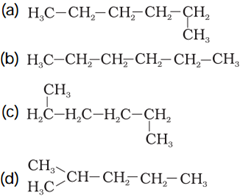Explanation: A branched chain hydrocarbon must contain some side chains which are bonded with parent carbon chain.
Q.24. Which among the following are unsaturated hydrocarbons?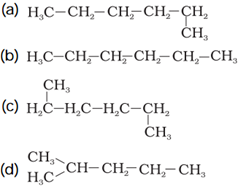(a) (i) and (iii)
(b) (ii) and (iii)
(c) (ii) and (iv)
(d) (iii) and (iv)
Explanation: Unsaturated hydrocarbons have multiple covalent bonds (double or triple bond) like alkene and alkyne.
Q.25. Which of the following does not belong to the same homologous series?
(a) $CH_{4}$
(b) $C_{2}H_{6}$
(c) $C_{3}H_{8}$
(d) $C_{4}H_{8}$
Explanation: Successive members of same homologous series are differ by $- CH_{2}$ unit. $CH_{4}, C_{2}H_{6}, C_{3}H_{8}$ belong to same series that is of alkane and differ by $─ CH_{2}$ unit but C4H8 does not belong to this.
Q.26.The name of the compound, $CH_{3} ─ CH_{2} ─ CHO$ is:
(a) Propanal
(b) Propanone
(c) Ethanol
(d) Ethanal
Explanation: Compound contains three carbon atoms so prop- would be root word and – CHO functional group so suffix will be –al. Hence name would be propane + al = propanal.
Q.27. The heteroatoms present in $CH_{3} ─ CH_{2} ─ O ─ CH_{2} ─ CH_{2}Cl$ are:
(i) Oxygen
(ii) Carbon
(iii) Hydrogen
(iv) Chlorine
(a) (i) and (ii)
(b) (ii) and (iii)
(c) (iii) and (iv)
(d) (i) and (iv)
Explanation: Atoms other than C and H in organic compound are called as heteroatoms.
Q.28. Which of the following represents saponification reaction?